# How to use the PRODUCT Function in Excel

The PRODUCT function is a mathematical operator function which multiplies the numbers. PRODUCT function excel returns product of all cell values taken in as arguments.
Syntax:

=PRODUCT (number1, [number2], ...)

Note: Logical values, empty cell & other texts in the arguments are ignored
Let’s understand this function using it in an example.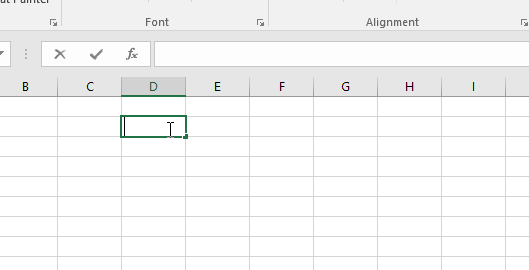As you can see in the above snapshot the PRODUCT function takes the product of all the values in the argument and returns the product in the cell.

Now we will understand it using cell references in the formula.

Here we have a list of values to take product of values.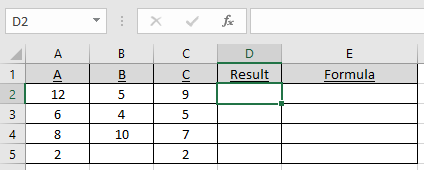Use the formula:

=PRODUCT(A2:C2)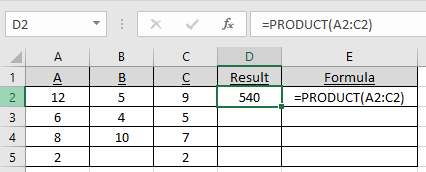As you can see the product of the numbers is cacluated using the PRODUCT function
Copy the formula in other cells using shortcut Ctrl + D.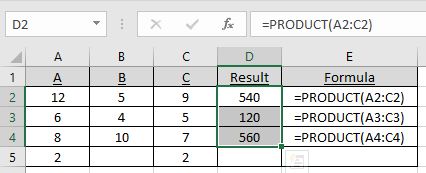You can multiply a number as argument with cell references.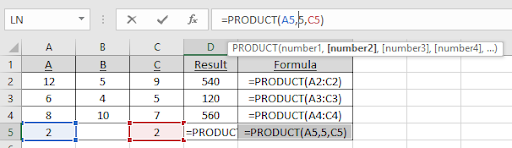Press Enter to get the product of numbers.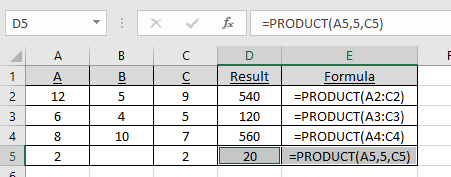As you can see, the function returns the product of all the values.

Use the PRODUCT function with other functions to see how it is used with other functions.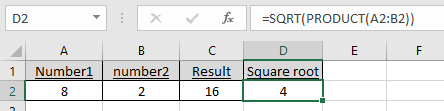As you can see the SQRT function with PRODUCT function.

Hope you understood how to use the PRODUCT function and referring cell in Excel. Explore more articles on Excel mathematical functions here. Please feel free to state your query or feedback for the above article.

Related Articles:

How to use the SUMPRODUCT Function in Excel

SUMPRODUCT with IF logic

Popular Articles:

How to use the VLOOKUP Function in Excel

How to use the COUNTIF function in Excel 2016

How to Use SUMIF Function in Excel

Terms and Conditions of use

The applications/code on this site are distributed as is and without warranties or liability. In no event shall the owner of the copyrights, or the authors of the applications/code be liable for any loss of profit, any problems or any damage resulting from the use or evaluation of the applications/code.